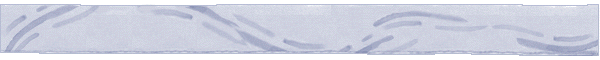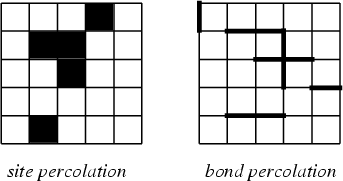Percolation Theory and Forest FiresTypes of Percolation

There are two types of percolation, depending how one views the lattice: site percolation and bond percolation.

In site percolation one views the lattice as a rectangular array of squares. Each square is populated by a dot, or is colored black below, with probabiility p (open square), otherwise closed with probability 1-p. A cluster is defined as set of neighboring squares that are open.

In bond percolation one views the lattice as a graph consisting of horizontal and vertical edges. Each lattice edge is open (boldfaced below) with probabiliy p, otherwise closed with probability 1-p. A cluster is defined as a lattice subgraph consisting of open edges.